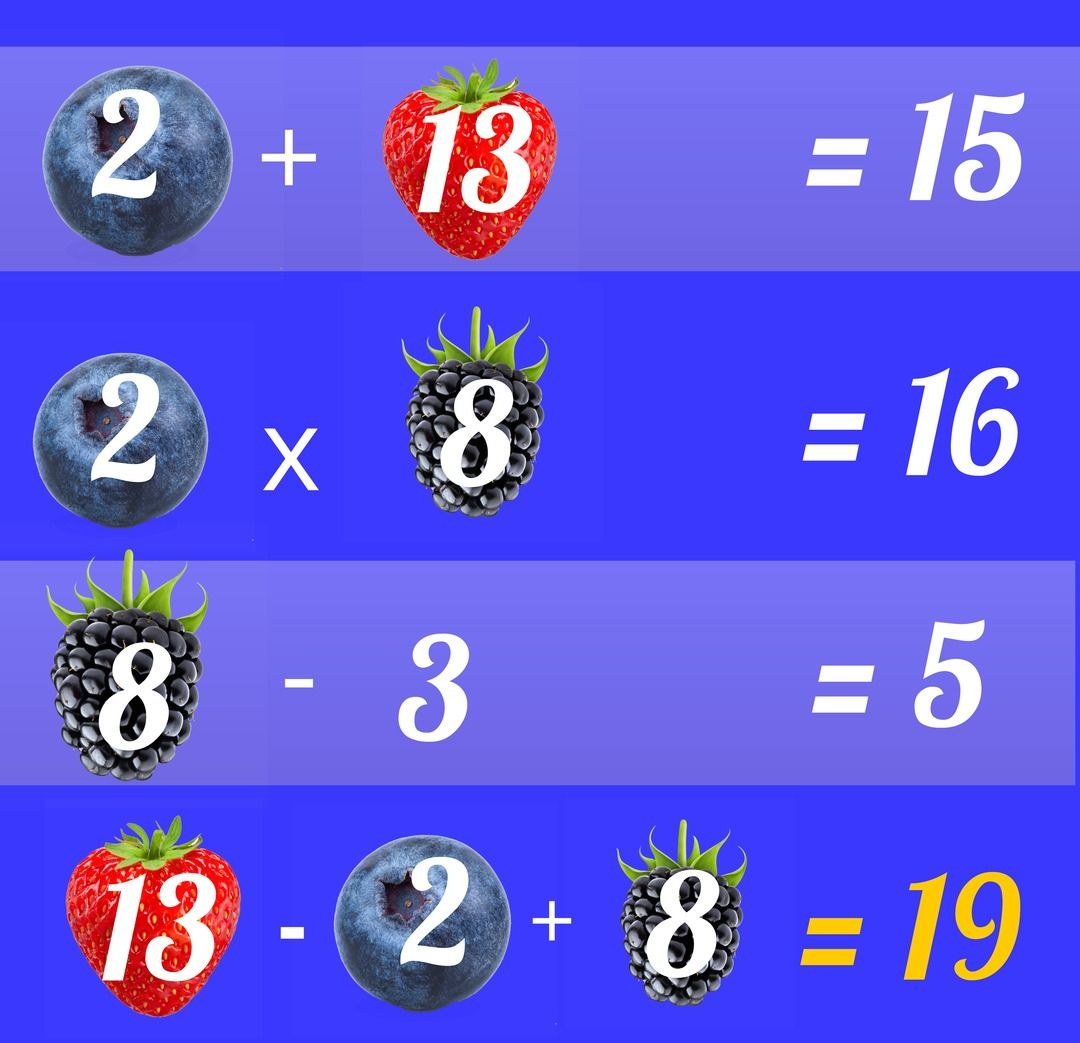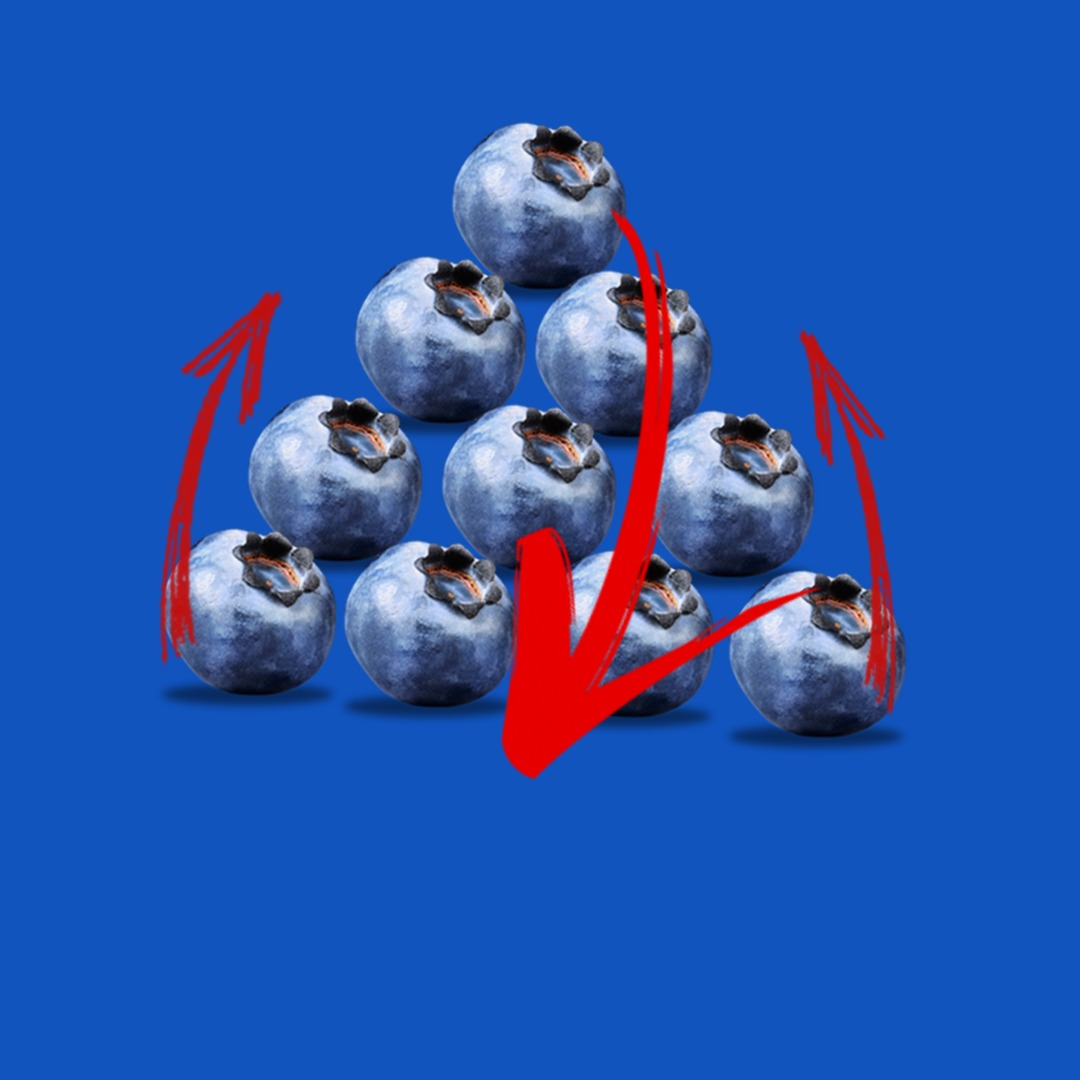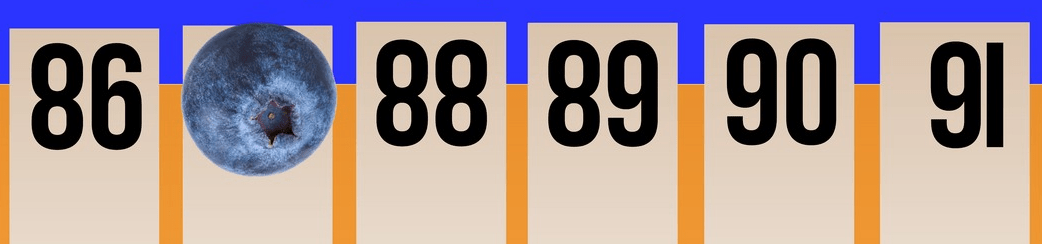Select Page

##### Missing Letter
R. The R stands for reverse on a manual
gearshift.
##### Missing Number
The answer is 66.

Each time you add the number by the next multiple of 3.

3 + 3 = 6
6 + 6 = 12
12 + 9 = 21
21 + 12 = 33
33 + 15 = 48
48 + 18 = 66
66 + 21 = 87

205

##### Berry Math
The Answer is 3.
Triangle is 6 and Star value is 3.
##### Missing Number
The answer is 45.
Difference between two consecutive numbers increases by 2 from the previous difference.
##### Unlock The Phone
The passcode is 164
##### April 27 answer
The answer is 32.

The solution to this problem is
(a+b) x 3 – a

(1 +2) x 3 -a
(3) x 3 – 1
9-1 =8

(7+6) x 3-7
13 x 3 – 7
39 – 7 = 32

##### Barry's Age
Barry’s mother was 23 when Barry was born. Now that Barry is 23, his mother is twice his age.
##### Train
2 minutes. The train traveling at 60 mph will cover one mile in a minute. So the engine of the train will clear the tunnel in one minute and it will take the last car another minute to clear the tunnel.
The time is 8:45
##### Most Occurring Digit
1. It appears 301 times
##### Cigarette
C) 10. Ben gets 8 cigarettes from the 32. Then he gets two more after he smokes the 8.
##### Handshake
The answer is 21.
##### 11
The answer is 1. At the very first we said
1 = 11.
##### How many berries
He has 3 berries.
##### Number wheel
4. The number across from each other is either its square of the square root
##### Hourglass
Step 1. Turn over both hourglasses.
Step 2. When the four minute glass runs out, turn it over.
Step 3. When the seven minute hourglass runs out. Turn it over.
Step 4. When the four minute timer runs out, turn over both timers. 8 minutes have passed and there is now one minute left in the 7 minute timer.
##### 7's
This is another orders of operations puzzle. Brackets, Exponents, Multiplication, Division, Addition and Subtraction

7 + {7/7} + {7X7} – 7
7+ 1 + 49 – 7 = 50

##### Writing 8's
He will have to write 8 20 times

8, 18, 28, 38, 48, 58, 68, 78, 80, 81, 82, 83, 84, 85, 86, 87, 88 (2), 89, 98

##### Sequence
21. The next number is found by adding the two previous numbers together. 0+1=1 1+1 =2
1+2=3 2+3 = 5 and so on.
##### What time?

5:01.  Each watch shows an increment of 42 minutes.

##### Buying & Selling Berries
\$3. He made \$1 on the first transaction and made \$2 on the second transaction. The first and second transactions are independent of each other.
##### Teresa
The answer is C) daughter. TO easily solve this you break the question into two parts and work the second part first. Who is my daughter’s mother? The answer is me. So the question now reads If Teresa’s daughter is me, what am I to Teresa? The answer is daughter.
##### How Many Birds

184 Berries

7 swans a swimming x 6 days          42
6 geese a laying x 7 days                   42
4 calling birds x 9 days                      36
3 French hens x 10 days                   30
2 turtle doves x 11 days                    22
1 partridge  x 12 days                        12

Total                                                      184

##### Boxes Of Berries

840 berries

The pattern is as follows the second box as twice as many berries as the first box. The third box has three times the berries as the second box. The fourth box has four time the berries as the third box. The fifth box will have five times the berries as the fourth,

##### The Number Wheel
The answer is 6. Each number when added to the number across from it will equal 21.
##### Jam Puzzle

The jam is 99 years old. There was never a year zero it went from 1BC to 1AD.

##### The \$1.10 riddle

The answer to the \$1.10 riddle the apple cost \$1.05  and the blueberry costs 5 cents.

Many will reply with an answer of 10, which is incorrect. Let us explain…

If the bat costs \$1.00 more than the ball and the total is \$1.10, then the ball must cost 5 cents and the bat must cost \$1.05.

If the ball were to cost ten cents then both together would cost \$1.20.

##### Nov 03 Math Puzzle

The answer is 13.

The blueberries are equal to 15 each
The strawberries are equal to 10 each
And the watermelon slices are equal to 5 each.

The equation at the bottom therefore is

10 + 15 ÷  5 = ?
The order of operations states that parenthesis are done first, then exponents, then multiplication and division and finally  addition and subtraction.

10 + (15 ÷ 5)
10 + 3 = 13.

##### 4 Litres Of Berries

Step 1. Fill the 5-Litre pot with blueberries

Step 2. Pour the berries from the 5-Litre pot into the 3-Litre pot until it is filled.

Step 3. Empty the 3-Litre pot and then pour the two remaining litres from the large pot into it. The 3-Litre pot now has 2 litres of berries.

Step 4. Fill the 5-Litre pot and then use it to top off the 3-Litre pot. You now have 4 litres  of berries in the 5-Litre pot.

##### Snail In The Well

At the end of each day the snail will be 1 foot higher in the well. On the 27th day he climbs from 26 feet to 29 feet and slides down to 27 feet. On the 28ty day he climbs to 30 feet and is out of the well.

##### Missing Number

6. Each number on the wheel and its opposite number equal 21. So 15 plus 6 equals 21.

##### September 29 Answer##### Are You A Genius

The answer is 6.

To get the number after the equals sign you have to multiply the the number before the equals sign by the number below.  8×7=56  7×6 =42 6×5 = 30 and so on. So 3×2 = 6

##### Doubling Berries

The field was full on day 99. The wording of the question along with the numbers 100 and one half lead many people to say day 50.

##### 100 Bill Anwer

\$100. The man steals the \$100 and returns it. What the owner loses is the \$70 in goods and \$30 in change.

##### Fruit Math##### Two Mothers and Two Daughters Answer

The two mothers and two daughters are only three people. There is a grandmother, a mother and a daughter.

The Grandmother and Mother make up the two mothers.

The Mother and the Daughter make up the two daughters.

##### Triangle Puzzle

There are 13 triangles.

##### My Father's Son answer

The answer to this puzzle is the man’s son. The easiest way to solve this is to answer  the last part of the question first. Who is my father’s son if I have no brothers? It’s me.  So replace my father’s son with the word me. Brothers and sisters I have none, but this man’s father is me. And there you have the answer.

##### Berry Triangle PuzzlePlace the top berry on the bottom.  Next move the two berries from the now second bottom row and place them on the now top row.

##### Weighing Blueberries Puzzle Answer

Divide the berries into three groups of three. Weight two of these groups and set the third group aside. If the one group is heavier than the other you know which group contains the heaviest berry. If both groups balance then the heaviest berry is in the third group.

Take the group with the heaviest berry and put two of the berries on the scale and set the third berry aside. If scales do not balance you know which berry is the heaviest. If the scales balance, then the third berry is the heaviest.

##### Berry Math Puzzle Answer.
ORDER OF OPERATIONS: Brackets, then Exponents, then Multiplication and Division in the order in which they occur, then Addition and Subtraction in the order in which they occur.
So: strawberry=5, blueberry=10, and raspberry=1
5+1×10
Multiplication is done before addition.. so 1×10=10
5+10=15
##### Berry Parking Lot

The trick of this puzzle is that you are viewing the numbers upside down. When you view them correctly you see the pattern 86, ?, 88, 89, 90 ,and 91. The berry is covering parking space 87.